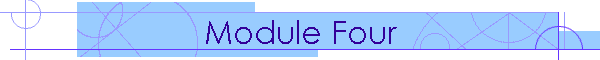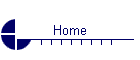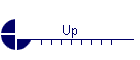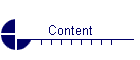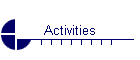Geometry and Spatial Reasoning

 Content Activities Day 1 (4 hours) Introduction Introduction to Cabri JuniorTM Software Inductive Reasoning Deductive Reasoning Pythagorean Theorem Four Proofs of the Pythagorean Theorem Tutorial on Irrational Numbers Day 2 (4 hours) Euclidean Geometry Axiomatic Systems Parallel Lines Land Ho! Constructions Day 3 (4 hours) Congruent Triangles Look Alikes Polygons Puzzling Polygons Similarity Patterns by Design Day 4 (4 hours) Transformations Reflections, Translations, Rotations Hole in One! Explorations with Patty Paper Tantalizing Tessellations Day 5 (4 hours) Transformations in Coordinate Plane Set the Sails! Dilations Shadows on the Wall Day 6 (4 hours) Right Triangle Trigonometry Tutorials on Special Right Triangles, Trigonometric Ratios, Finding Missing Angles of Rt. Triangles, Finding Missing Sides of Rt. Triangles Unit Circle Trigonometry Tutorial on Rationalizing Denominators